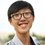# The must be a faster way - 1

If $p,q,r$ are the roots of the equation

$x^3-3px^2+3q^2x-r^3$

Prove that $p=q=r$.

Elementary proof:

$p+q+r=3p$

$pq+qr+rp=3q^2$

$pqr=r^3$

From the third equation,

$pq=r^2$

Substitute to the second equation,

$r^2+qr+rp=3q^2$

$r(p+q+r)=3q^2$

$3rp=3q^2$

$pr=q^2$

Substitute $pr=q^2$ into the third equation,

$q^3=r^3\implies q=r$

Again,

$pq=r^2$

$pr=r^2$

$p=r \implies p=q=r$

Can you find a better proof?Note by Christopher Boo
5 years, 11 months ago

This discussion board is a place to discuss our Daily Challenges and the math and science related to those challenges. Explanations are more than just a solution — they should explain the steps and thinking strategies that you used to obtain the solution. Comments should further the discussion of math and science.

When posting on Brilliant:

• Use the emojis to react to an explanation, whether you're congratulating a job well done , or just really confused .
• Ask specific questions about the challenge or the steps in somebody's explanation. Well-posed questions can add a lot to the discussion, but posting "I don't understand!" doesn't help anyone.
• Try to contribute something new to the discussion, whether it is an extension, generalization or other idea related to the challenge.

MarkdownAppears as
*italics* or _italics_ italics
**bold** or __bold__ bold
- bulleted- list
• bulleted
• list
1. numbered2. list
1. numbered
2. list
Note: you must add a full line of space before and after lists for them to show up correctly
paragraph 1paragraph 2

paragraph 1

paragraph 2

[example link](https://brilliant.org)example link
> This is a quote
This is a quote
    # I indented these lines
# 4 spaces, and now they show
# up as a code block.

print "hello world"
# I indented these lines
# 4 spaces, and now they show
# up as a code block.

print "hello world"
MathAppears as
Remember to wrap math in $$ ... $$ or $ ... $ to ensure proper formatting.
2 \times 3 $2 \times 3$
2^{34} $2^{34}$
a_{i-1} $a_{i-1}$
\frac{2}{3} $\frac{2}{3}$
\sqrt{2} $\sqrt{2}$
\sum_{i=1}^3 $\sum_{i=1}^3$
\sin \theta $\sin \theta$
\boxed{123} $\boxed{123}$

Sort by:

Put $x=r$ in the equation. Since $r$ is a root, we immediately get $pr=q^2$ (assuming $r\neq 0$). Also $pqr=r^3$. The rest follows from these two.

- 5 years, 11 months ago

Brilliant proof!!!

- 5 years, 11 months ago

Brilliant! @Abhishek Sinha

- 5 years, 11 months ago

we can assume that p=q . Which means that we are assuming p to be the repeated root of the given function . Differentiate the given cubic and let it be g(x). then substitute p in g(x) Since we have assumed p to be repeated root thus it will also be the root of g(x). on substituting p in the equation we will get p=q which concurs with our assumption.

- 5 years, 9 months ago

Indeed, I've found a much faster solution. Using the cubic formula, we get that

\begin{aligned}x&=\sqrt{\left(\frac{-(-3p)^3}{27(1)^3}+\frac{(-3p)(3q^2)}{6(1)^2}-\frac{(-r^3)}{2(1)}\right)+\sqrt{\left(\frac{-(-3p)^3}{27(1)^3}+\frac{(-3p)(3q^2)}{6(1)^2}-\frac{(-r^3)}{2(1)}\right)^2+\left(\frac{(3q^2)}{3(1)}-\frac{(-3p)^2}{9(1)^2}\right)^3}}\\&+\sqrt{\left(\frac{-(-3p)^3}{27(1)^3}+\frac{(-3p)(3q^2)}{6(1)^2}-\frac{(-r^3)}{2(1)}\right)-\sqrt{\left(\frac{-(-3p)^3}{27(1)^3}+\frac{(-3p)(3q^2)}{6(1)^2}-\frac{(-r^3)}{2(1)}\right)^2+\left(\frac{(3q^2)}{3(1)}-\frac{(-3p)^2}{9(1)^2}\right)^3}}\\&-\frac{(-3p)}{3(1)}\\&=\sqrt{p^3-\frac{3pq^2}2+\frac{r^3}2+\sqrt{\left(p^3-\frac{3pq^2}2+\frac{r^3}2\right)^2+\left(q^2-p^2\right)^3}}\\&+\sqrt{p^3-\frac{3pq^2}2+\frac{r^3}2-\sqrt{\left(p^3-\frac{3pq^2}2+\frac{r^3}2\right)^2+\left(q^2-p^2\right)^3}}\\&+p\end{aligned}

is one of $p$, $q$, and $r$. We can find the other roots by dividing out, and the rest of the proof is omitted.

- 5 years, 11 months ago

Honestly, why do you love to bash out all the problems so much?

- 5 years, 11 months ago